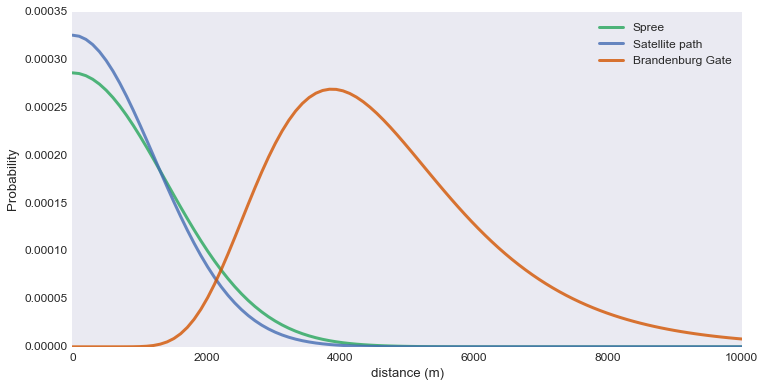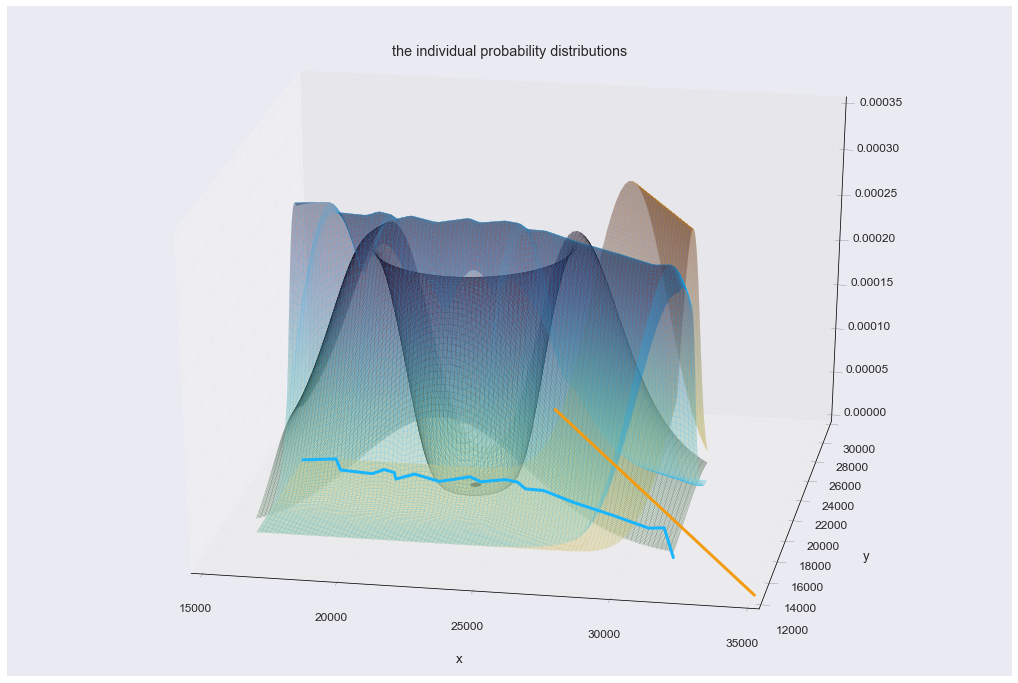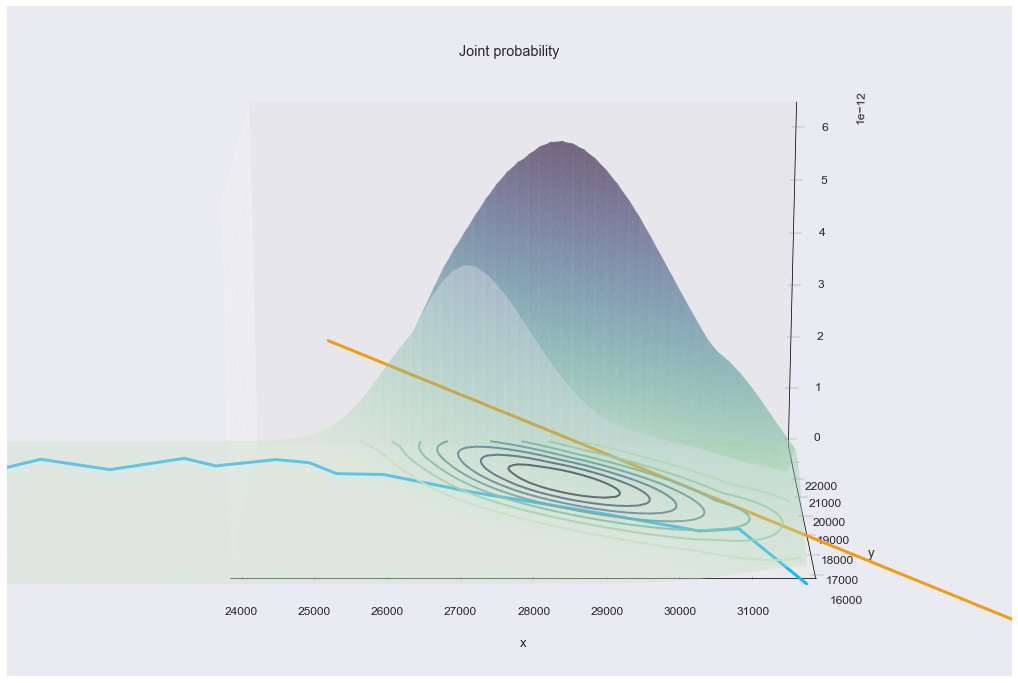# Zalando Challenge

## Introduction¶

I recently came across this little data challenge, which was posted by Zalando (one of the top fashion retailers in Europe) as a teaser for data scientists/analysts. The challenge is quite straightforward and is a good opportunity to show how to deal with this kind of analysis using the standard tools of python and the interactive notebook.

For data analysis, the community is in two minds between between python and R, but for spatial data it looks like the ecosystem has taken a bet on python. There are useful python libraries for all stages of a geoprocessing pipeline, from data handling (shapely, GDAL/ogr, pyproj, ...) to analysis (shapely, (geo)pandas, PySal, numpy/scipy, sklearn, etc) to plotting and visualisation (matplotlib, descartes, cartopy, pyQGIS). I've always found the last stage (visualisation) to be the most negelected, and trying to join up the data and analysis threads with interactive web-based visualisations (like and D3 and mapping libraries like leaflet) is usually a pain. The ipython notebook promises interactivity, and newer packages like Plotly and Bokeh are really pushing it along fast.

I will use Shapely for dealing with the geographic data, pyproj for projections and scipy for optimisation routines. It's nice to have interactive maps in the notebook, and for this I render the geojson geometries with google maps (although leaflet would be a fine choice too).

On to the challenge.

### Problem Statement¶

The Zalando Data Intelligence Team is searching for a new top analyst. We already know of an excellent candidate with top analytical and programming skills. Unfortunately, we don’t know her exact whereabouts but we only have some vague information where she might be. Can you tell us where to best send our recruiters and plot an easy to read map of your solution for them?

This is what we could extract from independent sources:

The candidate is likely to be close to the river Spree. The probability at any point is given by a Gaussian function of its shortest distance to the river. The function peaks at zero and has 95 of its total integral within ±2730 m.

A probability distribution centered around the Brandenburg Gate also informs us of the candidate’s location. The distribution’s radial profile is log-normal with a mean of 4700m and a mode of 3877m in every direction.

A satellite offers further information: with 95% probability she is located within 2400m distance of the satellite’s path (assuming a normal probability distribution)


6371km
Brandenburg Gate GPS coordinates

{
"geometry": {
"coordinates": [13.377689, 52.516288],
"type": "Point"
},
"type": "Feature"
}
Satellite path is a great circle path between coordinates

{
"geometry": {
"coordinates": [[13.39915, 52.590117], [13.553989, 52.437385]],
"type": "LineString"
},
"type": "Feature"
}
River Spree can be approximated as piecewise linear between the following coordinates:

{
"geometry": {
"coordinates": [[13.274099, 52.529198],
[13.29234, 52.531835], [13.298541, 52.522116],
[13.317349, 52.520569], [13.322434, 52.524877],
[13.329, 52.522788], [13.332075, 52.517056],
[13.340743, 52.522514], [13.356665, 52.517239],
[13.372158, 52.523063], [13.379453, 52.519198],
[13.392328, 52.522462], [13.399703, 52.520921],
[13.406054, 52.515333], [13.416354, 52.514863],
[13.435923, 52.506034], [13.461587, 52.496473],
[13.483216, 52.487641], [13.491456, 52.488739],
[13.503386, 52.464011]],
"type": "LineString"
},
"type": "Feature"
}

This information is given in the data file.

### Solution Outline¶

How to solve this problem?

In this case, the probability distributions are simple enough and we either have their parameters or can easily find them given the information. The probability distributions can be written as:

• Lognormal distribution centered on the Brandenburg Gate:
$$P_{BBgate}( {\mathbf{x}} \mid {\mathbf{x}}_{BBGate}, \mu_{BBgate}, \sigma_{BBgate}) = \log{\cal N}(\mu_{BBGate}, \sigma_{BBGate})$$
• Normal distribution of the shortest distance to a segment of the River Spree
$$P_{Spree}({\mathbf{x}} \mid \{{\mathbf{x}}_{0}\ldots{\mathbf{x}}_{N}\}_{Spree}, \mu_{Spree}, \sigma_{Spree}) = {\cal N}(\mu_{Spree}, \sigma_{Spree})$$
• Normal distribution centered on a satellite path, given as a great-circle arc between two endpoints
$$P_{sat}({\mathbf{x}} \mid \{{\mathbf{x}}_{0},{\mathbf{x}}_{1}\}_{sat}, \mu_{sat}, \sigma_{sat}) = {\cal N}(\mu_{sat}, \sigma_{sat})$$

These are independent observations of the probability of finding the person, and the solution will be the position ${\mathbf{x}}$ which maximises the total joint probability. Hopefully along the way we can shed some light on how to deal with spatial projections, distance calculations, optimisation and web mapping (all directly in a python notebook.

These are the imports I have used throughout the notebook and I've grouped them together for convenience.

In :
from __future__ import division
from functools import partial

import numpy as np
import jinja2

import matplotlib.pyplot as plt
from mpl_toolkits.mplot3d import Axes3D
from matplotlib import cm
import seaborn as sns

import scipy.stats as stats
import scipy.special as special
import scipy.optimize as opt

import shapely.geometry as geom
import shapely.ops as ops
import pyproj
from geojson import Feature, FeatureCollection
from geopy.geocoders import Nominatim

from IPython.display import display, HTML, Javascript
from plot_geoms import *
sns.set_context("paper", font_scale=1.5, rc={"figure.figsize": (18,12),
"lines.linewidth": 0.2,
"axes.grid": False,
"grid.linewidth": 0, })

geod = pyproj.Geod(ellps='WGS84')

%matplotlib inline

In :
js = """window.gmaps_ready = function() { console.log('initialised gmaps');} ;
if (typeof google === 'object' && typeof google.maps === 'object' && typeof google.maps.Map === 'function') { initialize(); } else {
$.getScript("https://maps.googleapis.com/maps/api/js?v=3.exp&callback=gmaps_ready"); }""" #display(Javascript(js)) #ignore  The coordinates of the Spree are given in the data file and we use them here to make a Shapely LineString object. The satellite path is given as the start and end coordinates of a segment of a great circle. In : sat_beg, sat_end = (13.39915, 52.590117), (13.553989,52.437385) bb_gate_coords = (13.377689, 52.516288) spree_coords = [(13.274099, 52.529198), (13.29234, 52.531835),(13.298541, 52.522116), (13.317349, 52.520569),(13.322434, 52.524877), (13.329, 52.522788),(13.332075, 52.517056), (13.340743, 52.522514),(13.356665, 52.517239), (13.372158, 52.523063),(13.379453, 52.519198), (13.392328, 52.522462),(13.399703, 52.520921), (13.406054, 52.515333),(13.416354, 52.514863), (13.435923, 52.506034),(13.461587, 52.496473), (13.483216, 52.487641),(13.491456, 52.488739), (13.503386, 52.464011)] spree = geom.LineString(spree_coords) satellite_path = geom.LineString([sat_beg, sat_end]) bb_gate = geom.Point(bb_gate_coords)  In : Feature(geometry=bb_gate)  Out: {"geometry": {"coordinates": [13.377689, 52.516288], "type": "Point"}, "properties": {}, "type": "Feature"} In : tpl = jinja2.Template(open('./gmap.tpl', 'r').read()) div_id = 'map-canvas-{}'.format("1") extra_css = "#{div_id} {{height: 600px; width:100%; margin:0; padding:0;}}".format(div_id=div_id) center, zoom = {"lng":13.45525234770495, "lat":52.51148144124993}, 12 spree_c, sat_c, bb_c = '#19B5FE', '#F39C12', '#2ECC71' feat_spree = Feature(geometry=spree, properties={'title':"River Spree", 'strokeColor': spree_c, 'strokeWeight':5, 'strokeOpacity':0.9}) feat_sat = Feature(geometry=satellite_path, properties={'title': "Satellite Path", 'strokeColor': sat_c, 'strokeWeight':5, 'strokeOpacity':0.9}) feat_bb_gate = Feature(geometry=bb_gate, properties={'strokeColor': bb_c, 'title':'Brandenburg Gate'}) tpl_html = tpl.render(div_id=div_id, suffix=div_id.replace('-', '_'), center=center, zoom=zoom, extra_css=extra_css, feature_collection=FeatureCollection([feat_spree, feat_sat, feat_bb_gate])) display(HTML(tpl_html))  The probability distribution around the Brandenburg Gate is lognormal in every direction. A lognormal distribution is a continuous probability distribution of a random variable (mean$\mu$and variance$\sigma$) whose log is normally distributed. The mean of a lognormal is given by$\exp(\mu + \sigma^{2}/2)$and the mode by$\exp(\mu - \sigma^{2})$. Knowing the mean($4700m$) and mode($3877m$), we can solve for$\mu$and$\sigma$: $$\begin{eqnarray} \sigma &=& \sqrt{\frac{2}{3} \left( \log(mean) - \log(mode)\right)} &=& 0.358237211939\\ \mu &=& \frac{2 \log(mean) + \log(mode)}{3} &=& 8.39115083769 \end{eqnarray}$$ In : bbgate_mean, bbgate_mode = 4700, 3877 bbgate_mu = (2.0*np.log(bbgate_mean) + np.log(bbgate_mode))/3.0 bbgate_sigma = np.sqrt((2.0/3.0)*(np.log(bbgate_mean) - np.log(bbgate_mode))) print bbgate_mu, bbgate_sigma  8.39115083769 0.358237211939  For a normal distribution, the probability that an observation is within a given number of standard deviations is $$\mu \pm x \sigma = {\mathrm{erf}}\left(\frac{x}{\sqrt{2}}\right)$$ and the commonly used 'two-standard deviations' is almost$95\%$likely: $$P(\mu - 2\sigma \leq x \leq \mu + 2\sigma) = {\mathrm{erf}}(2/\sqrt{2}) = 0.95449973610364158$$ With exactly$95\%$probability we are within almost$1.96$standard deviations: $$\sqrt(2) {\mathrm{erfinv}}(0.95) = 1.95996398454$$ and so we can find the standard deviation of the normal probability distributions given the$95\%$confidence interval: $$\sigma = \frac{x_{95\%} } {\sqrt{2} {\mathrm{erfinv}}(0.95)}$$ In : spree_sigma = 2730 / (special.erfinv(0.95) * np.sqrt(2)) sat_sigma = 2400 / (special.erfinv(0.95) * np.sqrt(2)) print spree_sigma, sat_sigma  1392.8827374 1224.51229662  And now we have all the parameters we need:$\mu_{BBGate} = 8.39115083769\sigma_{BBGate} = 0.358237211939\sigma_{Spree} = 1392.8827374\sigma_{Sat} = 1224.51229662$In : norm_spree = stats.norm(0, spree_sigma) norm_sat = stats.norm(0, sat_sigma) lognorm_bbgate = stats.lognorm(bbgate_sigma, scale=np.exp(bbgate_mu)) v = np.linspace(0, 10000, 100) lw=3 plt.figure(figsize=(12,6)) plt.plot(v, norm_spree.pdf(v), alpha=0.8, color='#26A65B', linewidth=lw, label='Spree') plt.plot(v, norm_sat.pdf(v), alpha=0.8, color='#446CB3', linewidth=lw, label='Satellite path') plt.plot(v, lognorm_bbgate.pdf(v), alpha=0.8, color='#D35400', linewidth=lw, label='Brandenburg Gate') plt.ylabel('Probability') plt.xlabel('distance (m)') _ = plt.legend()The coordinates we are given in the problem are standard latitude, longitude geographic coordinates (EPGSG:4326), on an oblate spheroid with major radius$R=6378137m$at the equator. Finding the distances between points in geographic coordinates involves spherical distance calculations (great-circle or Vincenty), which we would prefer to avoid. It is convenient to project the points into an orthogonal coordinate system, for which EPSG:3068 looks suitable. In this coordinate system, the distance unit is metres and our standard euclidean distance routines will provide good approximations to the spherical calculations (as long as the distances are fairly small). For handling the geographic coordinates we will use Shapely which is based on the geos library. Shapely does not do coordinate system transformation and all operations assume the features are in the same Cartesian plane. The coordinate system transformations are handled by pyproj. In : # create a transform function using pyproj Projections p_4326, p_3068 = pyproj.Proj(init='epsg:4326'), pyproj.Proj(init='epsg:3068') p_4326_3068 = partial(pyproj.transform, p_4326, p_3068) p_3068_4326 = partial(pyproj.transform, p_3068, p_4326) bb_gate_t = ops.transform(p_4326_3068, bb_gate) spree_t = ops.transform(p_4326_3068, spree) satellite_path_t = ops.transform(p_4326_3068, satellite_path)  In : # Here we compute the distance by geodetic or great circle methods (using pyproj) in epsg:4326 # and compare with the cartesian distance in epsg:3068 # two points in Berlin p1 = geom.base.geom_from_wkt('POINT (13.37927947157996 52.51928993794976)') p2 = geom.base.geom_from_wkt('POINT (13.274099 52.529198)') print 'EPSG:4326 spherical distance |p2-p1|', geod.inv(p1.x, p1.y, p2.x, p2.y) print 'EPSG:3068 cartesian distance |p2-p1|', ops.transform(p_4326_3068, p1).distance(ops.transform(p_4326_3068, p2))  EPSG:4326 spherical distance |p2-p1| 7223.53740724 EPSG:3068 cartesian distance |p2-p1| 7223.53619589  We see that cartesian distances in EPSG:3068 are a very good approximation to the spherical distances, at least for areas covering Berlin, and we can safely use the EPSG:3068 projection. In the challenge statement, Zalando provide a simple transformation to an orthogonal coordinate system, which should be a good approximation to EPSG:3068- because of the convenience of the pyproj transformations I will use them exclusively. This is how we compute the joint probability distribution: In : def compute_prob(p, origin_geom=None, prob_dist=None): return prob_dist.pdf(p.distance(origin_geom)) def prob(x,y): p = geom.Point(x, y) p_bb_gate = compute_prob(p, origin_geom=bb_gate_t, prob_dist=lognorm_bbgate) p_sat = compute_prob(p, origin_geom=satellite_path_t, prob_dist=norm_sat) p_spree = compute_prob(p, origin_geom=spree_t, prob_dist=norm_spree) return (p_bb_gate * p_spree * p_sat)  Let's make a grid and take a look at the individual probability distributions: In : N=100 (minx, miny, maxx, maxy) = spree_t.bounds xs, ys = np.linspace(minx, maxx, N), np.linspace(miny, maxy, N) xg, yg = np.meshgrid(xs, ys)  In : fig = plt.figure(figsize=(18,12)) ax = fig.add_subplot(111,projection="3d") alpha=0.4 offset = 0 cmap = sns.cubehelix_palette(12, start=0.5, rot=-.65, as_cmap=True) for (g, prob_dist, color) in [(satellite_path_t, norm_sat, sat_c), (spree_t, norm_spree, spree_c), (bb_gate_t, lognorm_bbgate, 'black')]: zg_geom = np.array([compute_prob(geom.Point(x,y), origin_geom=g, prob_dist=prob_dist) for x,y in zip(np.ravel(xg), np.ravel(yg))]) zg_geom = zg_geom.reshape(xg.shape) ax.plot_surface(xg, yg, zg_geom, rstride=1, cstride=1, alpha=alpha, cmap=cmap,lw=0.2, edgecolor=color, antialiased=True) plot_geom(spree_t, ax=ax, color=spree_c, linewidth=3) plot_geom(satellite_path_t, ax=ax, color=sat_c, linewidth=3) plot_geom(bb_gate_t, ax=ax, size=200, color='black') ax.view_init(elev=28) ax.azim = 360-90+10 ax.set_xlim3d(xmax=35000) #_ = ax.set_zlim(zmin=offset) _ = ax.set_xlabel('x') _ = ax.set_ylabel('y') _ = plt.title('the individual probability distributions') #_ = plt.tight_layout()If we look at the this on a map we see there is only a small area where all 3 probability distributions have a significant overlap. The polygons below extend out to the$95\%$confidence interval of each distribution. In : # 95% confidence intervals level = 0.975 dilated_spree = spree_t.buffer(norm_spree.ppf(level), cap_style=3, resolution=32) dilated_sat = satellite_path_t.buffer(norm_sat.ppf(level), cap_style=3, resolution=32) dilated_bb_gate = bb_gate_t.buffer(lognorm_bbgate.ppf(level), cap_style=1, resolution=32) overlap = dilated_spree.intersection(dilated_sat).intersection(dilated_bb_gate) feat_overlap = Feature(geometry=ops.transform(p_3068_4326, overlap), properties={'strokeOpacity': 0.7, 'strokeColor': '#F1A9A0', 'fillColor': '#F1A9A0', 'zIndex':10, 'fillOpacity':0.4, 'title': 'Area of Overlap 95% confidence'}) feat_dilated_spree = Feature(geometry=ops.transform(p_3068_4326, dilated_spree), properties={'strokeOpacity': 0.7, 'strokeColor': spree_c, 'fillColor':spree_c, 'fillOpacity':0.05, 'zIndex':9}) feat_dilated_sat = Feature(geometry=ops.transform(p_3068_4326, dilated_sat), properties={'strokeOpacity': 0.7, 'strokeColor': sat_c, 'fillColor': sat_c, 'zIndex':9, 'fillOpacity':0.05}) feat_dilated_bbgate = Feature(geometry=ops.transform(p_3068_4326, dilated_bb_gate), properties={'strokeOpacity': 0.7, 'strokeColor': bb_c, 'fillColor':bb_c, 'zIndex':9, 'fillOpacity':0.05}) tpl = jinja2.Template(open('./gmap.tpl', 'r').read()) div_id = 'map-canvas-{}'.format("2") extra_css = "#{div_id} {{height: 600px; width:100%; margin:0; padding:0;}}".format(div_id=div_id) center, zoom = {"lng":13.45525234770495, "lat":52.51148144124993}, 11 tpl_html = tpl.render(div_id=div_id, suffix=div_id.replace('-', '_'), center=center, zoom=zoom, extra_css=extra_css, feature_collection=FeatureCollection([feat_overlap, feat_dilated_spree, feat_dilated_sat, feat_dilated_bbgate])) display(HTML(tpl_html))  What does the joint probability look like? In : def draw_prob_3d(alpha_surface=0.4): fig = plt.figure(figsize=(18,12)) ax = fig.gca(projection='3d') ax.view_init(elev=18) offset = 0 zg = np.array([(prob(x,y)) for x,y in zip(np.ravel(xg), np.ravel(yg))]) zg = zg.reshape(xg.shape) ax.plot_surface(xg, yg, zg, rstride=1, cstride=1, alpha=alpha_surface, cmap=cmap,lw=0., edgecolor='#ABB7B7', antialiased=True) cset = ax.contour(xs, ys, zg, zdir='z', linewidths=(2,), alpha=0.95, offset=offset, cmap=cmap) ax.set_zlim(zmin=offset) ax.set_xlabel('x') ax.set_ylabel('y') ax.azim = 270 plot_geom(spree_t, ax=ax, linewidth=3, color=spree_c, offset=offset) plot_geom(satellite_path_t, ax=ax, linewidth=3, color=sat_c, offset=offset) #plot_geom(geom.Point(p_opt_t), ax=ax, color='red', size=100) _ = ax.set_xlim3d(24000, maxx) _ = ax.set_ylim3d(16000, maxy) _ = plt.title("Joint probability") return ax ax = draw_prob_3d()It looks like we have only 1 candidate for the most likely position. Since we already have a grid its straighforward to find the maximum. There are a couple of options in scipy- the grid search method is likely to be slow, but very reliable: scipy.optimize.brute. If we are sure there is only one maximum, we could choose a starting point (close enough to the position of the maximum) and call scipy.optimize.fmin. The scipy.optimize.differential_evolution global optimisation routine seems to do a very good job at finding the most likely position given the full bounds and this method is fast too, converging after$9$iterations in$170ms\$. We explicity call fmin to polish off this approximate result.

In :
%%time
res = opt.differential_evolution(lambda x: -prob(x, x), [(minx,maxx), (miny,maxy)],
maxiter=1000, polish=False)
print res
print ops.transform(p_3068_4326, geom.Point(res.x))

p_opt_t = opt.fmin(lambda x: -prob(x, x), res.x, maxiter=1000, ftol=1e-10)
print p_opt_t

p_opt = ops.transform(p_3068_4326, geom.Point(p_opt_t, p_opt_t))
print p_opt

    nfev: 390
success: True
fun: -6.3374835291765688e-12
x: array([ 28454.15708622,  20489.13953649])
message: 'Optimization terminated successfully.'
nit: 12
POINT (13.45536641235914 52.51139342727278)
Optimization terminated successfully.
Current function value: -0.000000
Iterations: 56
Function evaluations: 109
[ 28446.43610387  20498.95190559]
POINT (13.45525234640474 52.51148144197305)
CPU times: user 179 ms, sys: 1.31 ms, total: 180 ms
Wall time: 188 ms

In :
ax = draw_prob_3d(alpha_surface=0.25)
plot_geom(geom.Point(p_opt_t), ax=ax, color='red', size=150)